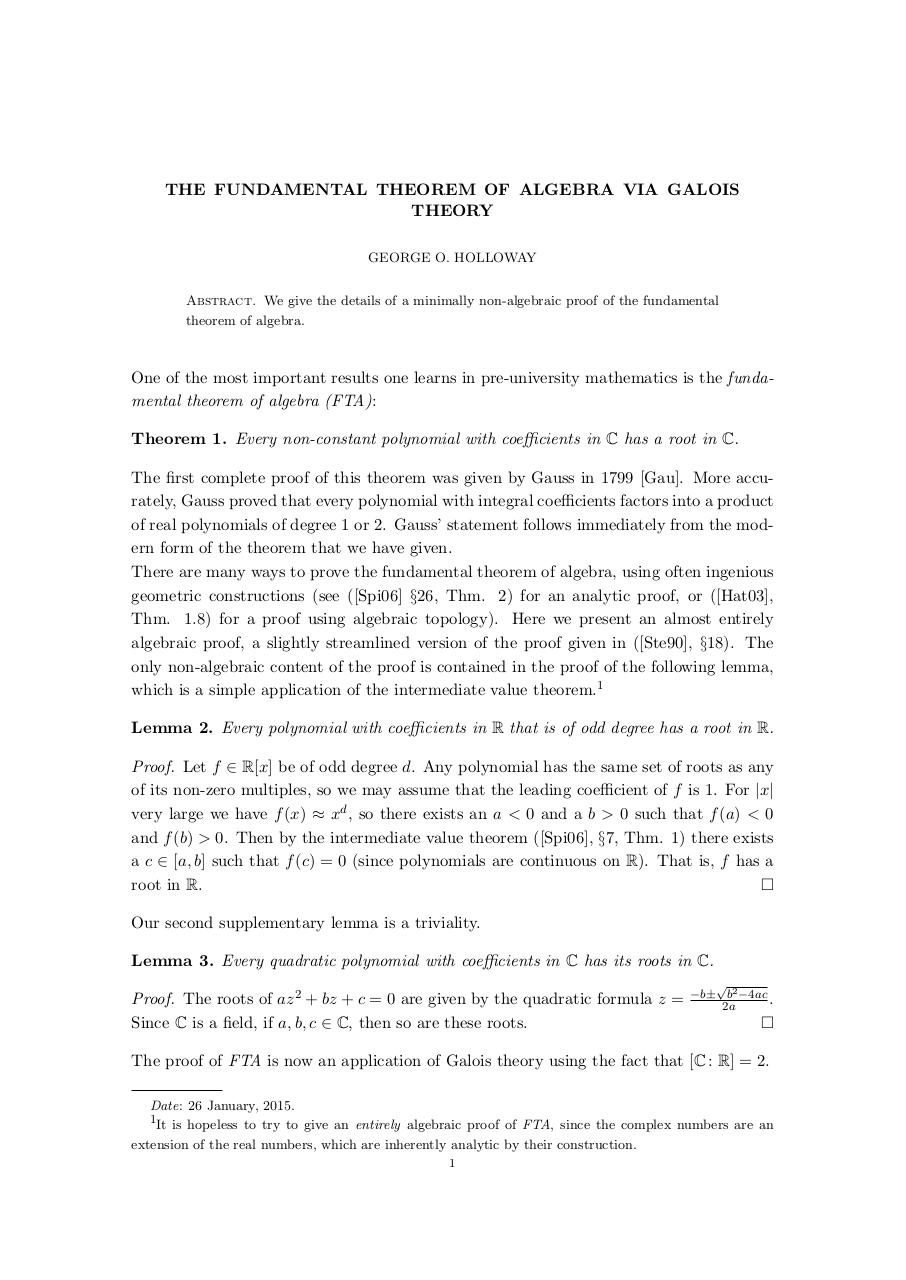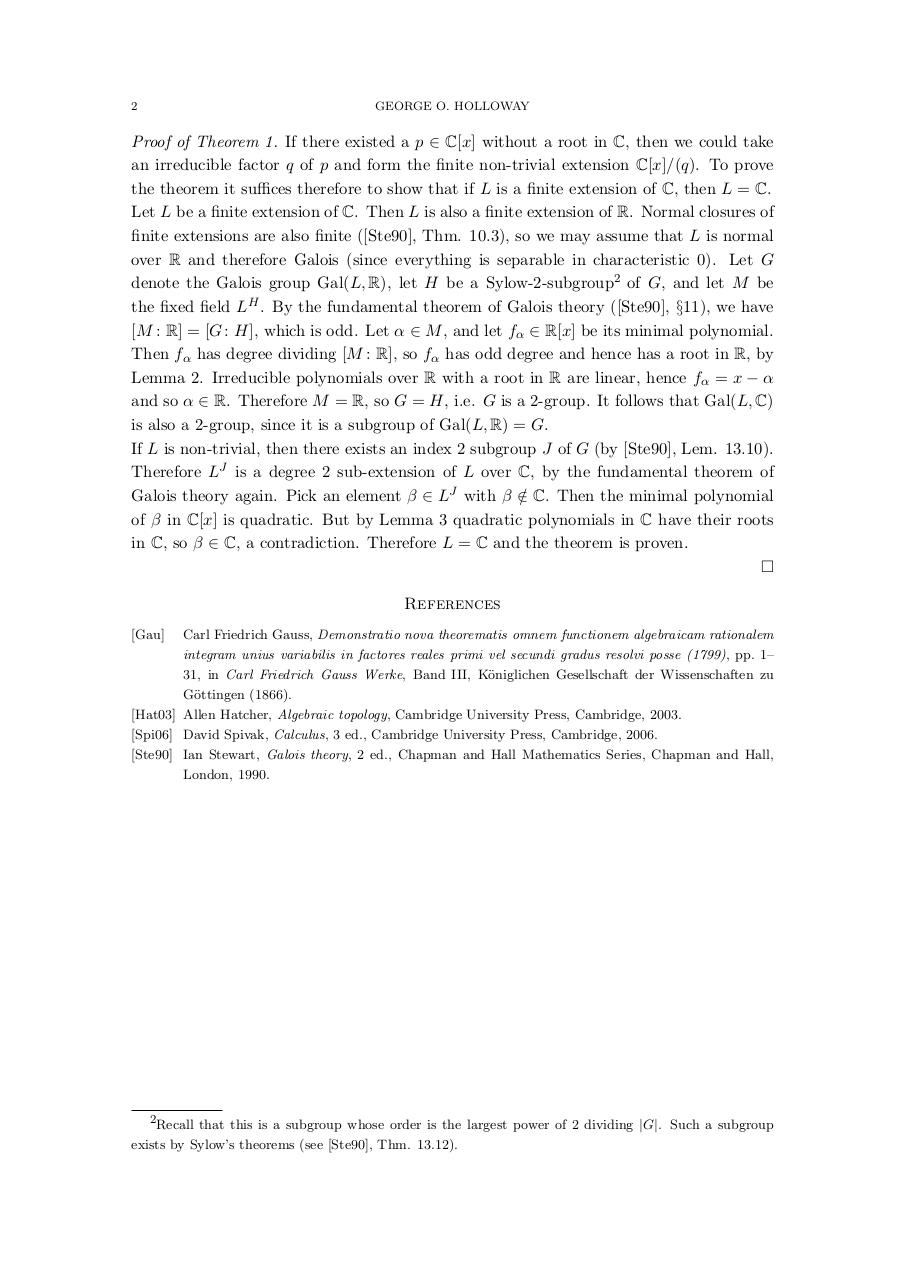# FundThmAlgebraGalois .pdf

### File information

Original filename: FundThmAlgebraGalois.pdf

This PDF 1.5 document has been generated by TeX / pdfTeX-1.40.16, and has been sent on pdf-archive.com on 14/07/2016 at 22:05, from IP address 86.173.x.x. The current document download page has been viewed 497 times.
File size: 175 KB (2 pages).
Privacy: public file

FundThmAlgebraGalois.pdf (PDF, 175 KB)

### Document preview

THE FUNDAMENTAL THEOREM OF ALGEBRA VIA GALOIS
THEORY
GEORGE O. HOLLOWAY
Abstract. We give the details of a minimally non-algebraic proof of the fundamental
theorem of algebra.

One of the most important results one learns in pre-university mathematics is the fundamental theorem of algebra (FTA):
Theorem 1. Every non-constant polynomial with coefficients in C has a root in C.
The first complete proof of this theorem was given by Gauss in 1799 [Gau]. More accurately, Gauss proved that every polynomial with integral coefficients factors into a product
of real polynomials of degree 1 or 2. Gauss’ statement follows immediately from the modern form of the theorem that we have given.
There are many ways to prove the fundamental theorem of algebra, using often ingenious
geometric constructions (see ([Spi06] §26, Thm. 2) for an analytic proof, or ([Hat03],
Thm. 1.8) for a proof using algebraic topology). Here we present an almost entirely
algebraic proof, a slightly streamlined version of the proof given in ([Ste90], §18). The
only non-algebraic content of the proof is contained in the proof of the following lemma,
which is a simple application of the intermediate value theorem.1
Lemma 2. Every polynomial with coefficients in R that is of odd degree has a root in R.
Proof. Let f ∈ R[x] be of odd degree d. Any polynomial has the same set of roots as any
of its non-zero multiples, so we may assume that the leading coefficient of f is 1. For |x|
very large we have f (x) ≈ xd , so there exists an a &lt; 0 and a b &gt; 0 such that f (a) &lt; 0
and f (b) &gt; 0. Then by the intermediate value theorem ([Spi06], §7, Thm. 1) there exists
a c ∈ [a, b] such that f (c) = 0 (since polynomials are continuous on R). That is, f has a
root in R.

Our second supplementary lemma is a triviality.
Lemma 3. Every quadratic polynomial with coefficients in C has its roots in C.
Proof. The roots of az 2 + bz + c = 0 are given by the quadratic formula z =
Since C is a field, if a, b, c ∈ C, then so are these roots.

−b± b2 −4ac
.
2a



The proof of FTA is now an application of Galois theory using the fact that [C : R] = 2.
Date: 26 January, 2015.
1It is hopeless to try to give an entirely algebraic proof of FTA, since the complex numbers are an
extension of the real numbers, which are inherently analytic by their construction.
1

2

GEORGE O. HOLLOWAY

Proof of Theorem 1. If there existed a p ∈ C[x] without a root in C, then we could take
an irreducible factor q of p and form the finite non-trivial extension C[x]/(q). To prove
the theorem it suffices therefore to show that if L is a finite extension of C, then L = C.
Let L be a finite extension of C. Then L is also a finite extension of R. Normal closures of
finite extensions are also finite ([Ste90], Thm. 10.3), so we may assume that L is normal
over R and therefore Galois (since everything is separable in characteristic 0). Let G
denote the Galois group Gal(L, R), let H be a Sylow-2-subgroup2 of G, and let M be
the fixed field LH . By the fundamental theorem of Galois theory ([Ste90], §11), we have
[M : R] = [G : H], which is odd. Let α ∈ M , and let fα ∈ R[x] be its minimal polynomial.
Then fα has degree dividing [M : R], so fα has odd degree and hence has a root in R, by
Lemma 2. Irreducible polynomials over R with a root in R are linear, hence fα = x − α
and so α ∈ R. Therefore M = R, so G = H, i.e. G is a 2-group. It follows that Gal(L, C)
is also a 2-group, since it is a subgroup of Gal(L, R) = G.
If L is non-trivial, then there exists an index 2 subgroup J of G (by [Ste90], Lem. 13.10).
Therefore LJ is a degree 2 sub-extension of L over C, by the fundamental theorem of
Galois theory again. Pick an element β ∈ LJ with β ∈
/ C. Then the minimal polynomial
of β in C[x] is quadratic. But by Lemma 3 quadratic polynomials in C have their roots
in C, so β ∈ C, a contradiction. Therefore L = C and the theorem is proven.

References
[Gau]

Carl Friedrich Gauss, Demonstratio nova theorematis omnem functionem algebraicam rationalem
integram unius variabilis in factores reales primi vel secundi gradus resolvi posse (1799), pp. 1–
31, in Carl Friedrich Gauss Werke, Band III, K¨
oniglichen Gesellschaft der Wissenschaften zu

ottingen (1866).
[Hat03] Allen Hatcher, Algebraic topology, Cambridge University Press, Cambridge, 2003.
[Spi06] David Spivak, Calculus, 3 ed., Cambridge University Press, Cambridge, 2006.
[Ste90] Ian Stewart, Galois theory, 2 ed., Chapman and Hall Mathematics Series, Chapman and Hall,
London, 1990.

2Recall that this is a subgroup whose order is the largest power of 2 dividing |G|. Such a subgroup

exists by Sylow’s theorems (see [Ste90], Thm. 13.12).